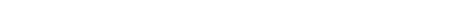### 12.5 Color models

Three color models are defined by default: RGB, HSV and GRAY. RGB and HSV allow specification of any color within conventional color space using three orthogonal coordinate axes, while gray restricts colors to one hue between white and black.All color models contain an optional alpha component, though this is used only on Mac OS X/Cocoa.

###### Color models defined by defaultModel

Name

Component: Range

RGB

Red Green Blue

RED (0.0 to 1.0)

GREEN (0.0 to 1.0)

BLUE (0.0 to 1.0)

ALPHA (0.0 to 1.0)

HSV

Hue Saturation Value

HUE (0.0 to 5.99999)

SATURATION (0.0 to 1.0)

VALUE (0.0 to 1.0)

ALPHA (0.0 to 1.0)

GRAY

Gray

GRAY (0.0 to 1.0)

ALPHA (0.0 to 1.0)

The Hue value in HSV is mathematically in the open interval [0.0 6.0). All values must be specified in floating point values.

You can convert color-specs between models using the available ``` ensure-<``` model ``` >``` functions. For example:

`(setf green (make-rgb 0.0 1.0 0.0) `
`    => #(:RGB 0.0 1.0 0.0))`
`(eq green (ensure-rgb green)) => T `
`(ensure-hsv green) => #(:HSV 3.0 0.0 1.0)`
`(eq green (ensure-hsv green) => NIL`
`(ensure-rgb (ensure-hsv green)) => #(:RGB 0.0 1.0 0.0)`
`(eq green (ensure-rgb (ensure-hsv green))) => NIL`

Of course, information can be lost when converting to GRAY:

`(make-rgb 0.3 0.4 0.5) => #(:RGB 0.3 0.4 0.5)`
`(ensure-gray (make-rgb 0.3 0.4 0.5)) `
`    => #(:GRAY 0.39999965)`
`(ensure-rgb (ensure-gray `
`             (make-rgb 0.3 0.4 0.5)))`
`       => #(:RGB 0.39999965 0.39999965 0.39999965)`

There is also ``` ensure-color``` which takes two color-spec arguments. It converts if necessary the first argument to the same model as the second. For example:

`(ensure-color (make-gray 0.3) green) `
`     => #(:RGB 0.3 0.3 0.3)`

``` ensure-model-color``` takes a model as the second argument. For example:

`(ensure-model-color (make-gray 0.3) :hsv)`
`     => #(:HSV 0 1.0 0.3)`

The function colors= compares two color-spec objects for color equality.

Conversion to pixel values is done by ``` convert-color``` .

LispWorks CAPI User Guide (Macintosh version) - 8 Apr 2005